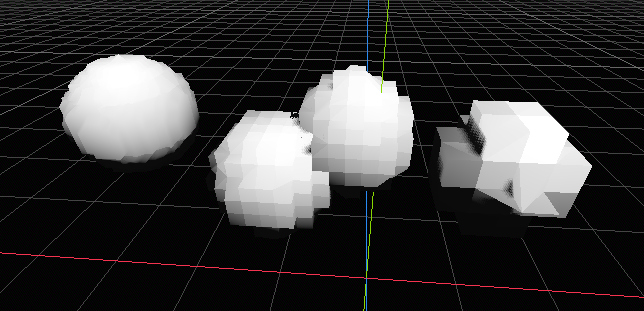# global vertex snapping with 3dresolution scaling

This shader converts all the vertex coordinates to global space using the object’s transform, then snaps every vertex to the nearest global position based on the “res” value which is a 3d scale of the implied resolution in global space. This was made in Godot 4.0.3.

``````// created by zodiepupper and xlinka (https://github.com/xlinka)
uniform float res;
uniform vec4 albedo;

void vertex() {
vec3 scale = vec3(length(MODEL_MATRIX), length(MODEL_MATRIX), length(MODEL_MATRIX));
mat4 rot_matrix = mat4(normalize(MODEL_MATRIX), normalize(MODEL_MATRIX), normalize(MODEL_MATRIX), vec4(0.0, 0.0, 0.0, 1.0));
mat4 scale_matrix = mat4(vec4(scale.x, 0.0, 0.0, 0.0),
vec4(0.0, scale.y, 0.0, 0.0),
vec4(0.0, 0.0, scale.z, 0.0),
vec4(0.0, 0.0, 0.0, 1.0));
vec4 world_pos = MODEL_MATRIX * vec4(VERTEX, 1.0);
world_pos.xyz = round(world_pos.xyz * res) / res;
vec4 local_pos = inverse(MODEL_MATRIX) * world_pos;

VERTEX = (rot_matrix * scale_matrix * local_pos).xyz;
}

void fragment() {
ALBEDO = albedo.rgb;
}``````
###### Tags
snap, vertexThe shader code and all code snippets in this post are under MIT license and can be used freely. Images and videos, and assets depicted in those, do not fall under this license. For more info, see our License terms.

### Vertex Mesh Rotator

Subscribe
Notify of1 Comment
Inline Feedbacks